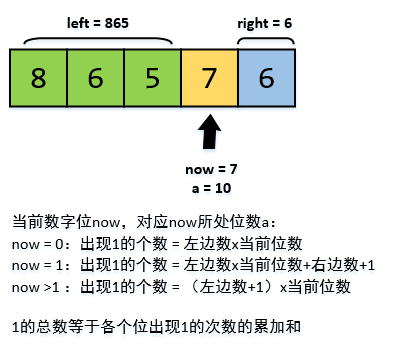### 题目

The task is simple: given any positive integer N, you are supposed to count the total number of 1's in the decimal form of the integers from 1 to N. For example, given N being 12, there are five 1's in 1, 10, 11, and 12.

Input Specification:
Each input file contains one test case which gives the positive N (≤2​30​​ ).

Output Specification:
For each test case, print the number of 1's in one line.

Sample Input:

12


Sample Output:

5


### 解析• N=345时，从1-345345个数中，百位数字可以出现0、1、2、3四种，每种百位数字都可以跟一个数字为1十位，而每种十位数字可以跟0-9这十种数字，所以从1~345345个数中，十位数字为1的数共有(3+1)×10=40个，故十位上的1共出现40次。
• N=305时，百位上数字依然可以出现0、1、2、3四种，但要注意，百位数字为3时，后面不能再跟数字为1十位，因为这样的数字已经大于305了，所以从1~305305个数中，十位数字为1的数共有3×10=30个，故十位上的1共出现30次。
• N=315时，百位上数字依然可以出现0、1、2、3四种，此时要注意，百位数字为3时，后面可以再跟数字为1十位，但这样的数字个位上只能出现0-56个数，即310、311、312、313、314、315，其他数字都会大于315，所以从1~315315个数中，十位数字为1的数共有3×10+(5+1)=36个，故十位上的1共出现36次。

• current=0：num = left × 10i

• current=1：num = left × 10i+ (right + 1)

• current>1：num = (left + 1) × 10i

• 其中10^i^就表示的是当前处理的是个位、十位、还是百位、千位.......

从右往左（从个位往高位）开始遍历，判断当前位置的字符：

• 若是0：则在当前位置可以出现1的次数为left * a次。

• 若是1：则在当前位置可以出现1的次数为left * a + right + 1

• 其他（2-9）：则在当前位置出现1 的次数为(left+1)*a

### 代码

#include <iostream>
using namespace std;

int main() {
int n, ans = 0, radix = 1, left, right, curr;
cin >> n;
left = n / (radix * 10); curr = n / radix % 10; right = n % radix;
if (curr == 0) ans += left * radix;
else if (curr == 1) ans += left * radix + right + 1;
else ans += (left + 1) * radix;